Science, Maths & Technology

### Become an OU student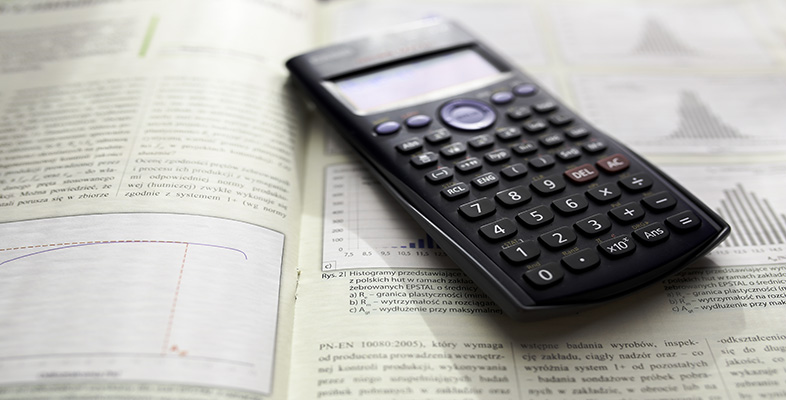Using a scientific calculator

Start this free course now. Just create an account and sign in. Enrol and complete the course for a free statement of participation or digital badge if available.

# 5 Scientific notation on your calculator

## Displaying numbers in scientific notation on your calculator

If the result of a calculation is a number greater than or equal to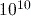(i.e.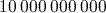), the calculator will automatically display the result using scientific notation. For example, calculating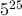gives the answer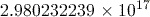,

which is displayed on the calculator screen as it is written here.

Small numbers are also automatically displayed using scientific notation. However, how small the number needs to be for this to happen depends on the mode the calculator is working in:

• ‘Norm 1’ mode uses scientific notation for any number less than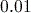but greater than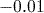.
• ‘Norm 2’ mode uses scientific notation for any number less than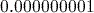but greater than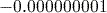.

‘Norm’ is short for ‘normal’.

In Activity 1 you will have already set your calculator to use Norm 2 mode, and we suggest that for the moment you continue to use this. To change the mode, use the key sequence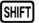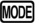(SETUP)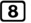(Norm) followed by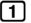(for Norm 1) or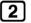(for Norm 2).

You can also set the calculator to always display results using scientific notation with a set number of significant figures using the key sequence(SETUP)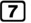(Sci) followed by the number of significant figures required, for example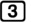. When your calculator is set in this fashion, the display indicator SCI is displayed at the top of the screen. To cancel such a setting, use one of the key sequences given above to return to a ‘Norm’ mode.